Home MonkeyNotes Printable Notes Digital Library Study Guides Study Smart Parents Tips College Planning Test Prep Fun Zone Help / FAQ How to Cite New Title Request

 2.8 The Exponential Limits If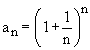then an is monotonic increasing and bounded, then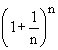and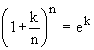If we put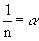, then as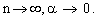Hence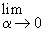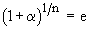andWe know that 'e' is an irrational number and 2 < 3 < 3. Also, we shall assume some results without proof.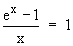and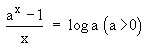The term log a on R.H.S. has base 'e' which need not be explicitly written. The students should note that the power of 'a' in the numerator viz 'x' must be repeated in the denominator and it must tend to zero. For this sometimes, the denominator has to be readjusted as follows.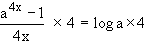.... (Since 4x ® 0 as x ® 0 ) Example 1 FindSolution : L =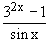=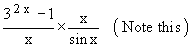=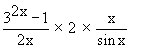=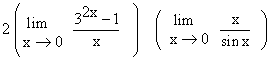= 2 (log 3) (1)=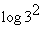= log 9 Example 2   FindSolution : L =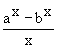=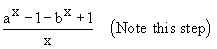==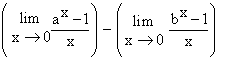= log a - log b = log (a / b) Index 2.1 Modulus 2.2 Inequalities 2.3 Limits Of Functions 2.4 Left Hand And Right Hand Limits 2.5 Theorems On The Algebra Of Limits 2.6 Evaluating Limits 2.7 Limits Of Trigonometric Functions 2.8 The Exponential Limits Chapter 3 Follow @Pinkmonkey_comYour browser does not support the IFRAME tag.